# Validating Various Input Data in FlashToday, almost everything on the web is based on user input. A contact form, a user registration form, a search box and so on. As a developer you can't just rely on the user to write everything as it's supposed to be. To be sure you always get the correct data from your users you will need to validate the input. Read on to find out how..

In this tutorial you will learn how various data is supposed to be structured and how to validate this data. Note that these methods may not be the best out there or the most complete solutions.

The following topics will be covered in this tutorial:

• Date and time validation
• E-mail validation
• Phone number validation
• International Standard Book Number (ISBN) validation
• International Bank Account Number (IBAN) validation
• Credit card number validation

Each topic is handled independently so you can follow the ones you are interested in.

## Final Result Preview

This is how our validator will look:

There are multiple fields with the sole purpose of demonstration. In a real scenario you will rarely have all these fields in a single form.

In this tutorial I will only cover the coding part. You will find the graphic interface in the source files. To follow along you will need Flash CS3+.

To better understand this tutorial you must have some basic knowledge about Regular Expressions and string functions. Please check this resource about regular expressions in ActionScript 3.0 if you don't feel comfortable with the syntax.

## Understanding the .fla File

Well it's not a very complicated interface. If you open the Validator.fla file in the begin folder of the Source download, you will find two frames: one locked, named ActionScript and another one called Interface.

The Interface layer is currently empty. There we will add our text boxes used for the data input.

On the ActionScript layer you will find this code:

 1 2 var validator:Validator = new Validator();  3 4 stage.addEventListener(FocusEvent.FOCUS_IN, register);  5 stage.addEventListener(FocusEvent.FOCUS_OUT, unregister);  6 7 function register(e:FocusEvent):void  8 {  9  e.target.addEventListener(Event.ENTER_FRAME, onFrameUpdate, false, 0, true);  10 }  11 function unregister(e:FocusEvent):void  12 {  13  e.target.removeEventListener(Event.ENTER_FRAME, onFrameUpdate);  14 }  15 16 function onFrameUpdate(e:Event):void  17 {  18  var input:String = e.target.text;  19  var valid:Boolean;  20   21  switch(e.target)  22  {  23   24  }  25   26  if(valid)  27  e.target.parent.validTick.gotoAndStop('valid');  28  else e.target.parent.validTick.gotoAndStop('invalid');  29 } 

The first line represents an instance of our future Validator class. We'll use a separate AS file to contain this class, so that it can be used in other projects.

We add event listeners to the stage for focus events. We will use these events to validate our input data only when a text field has focus just to avoid unnecessary calculations.

The onFrameUpdate function is called on every frame when a text field has focus to validate the data in it.

We'll add our future code in the switch statement. Everything else remains unchanged over this tutorial. In the library you will find a MovieClip called TextBox. This has an input TextField with an instance name of input_txt and another MovieClip called validTick with two frames labeled "valid" and "invalid".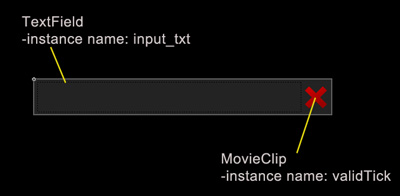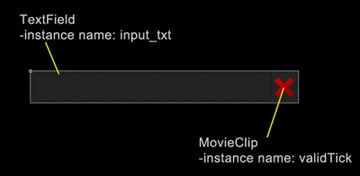Now let's get to the coding.

## Step 1: Preparations

First create a new ActionScript file and name it Validator.as

Paste or type in the following code:

 1 2 3 package  4 {  5  public class Validator  6  {  7 8  public function Validator()  9  {  10  trace('Validator created');  11  }  12  }  13 } 

This will be the framework of our Validator class. We will add methods to this class for each validation type we perform. Place this file in the same folder as the Validator.fla file.

## Step 2: Calendar Date Structure

The calendar date is one of the most frequently used data on the web. The structure varies from zone to zone. We will look at two main dates formats: United Kingdom version and United States version.

• The UK date is expressed as DD-MM-YYYY.
• The US date is expressed as MM-DD-YYYY.

In both cases DD stands for the day, MM stands for the month and YYYY stands for the year. It's also possible to use another character for the separator, like a slash.

## Step 3: Calendar Date Check

### Code

First of all we need to check to see if the date format is correct. Paste or type the following code in our Validator class just after the constructor:

 1 2 public function checkDate(date:String):Boolean  3 {  4  var month:String = "(0?[1-9]|1)";  5  var day:String = "(0?[1-9]|[0-9]|3)";  6  var year:String = "([1-9][0-9]{3})";  7  var separator:String = "([.\/ -]{1})";  8   9  var usDate:RegExp = new RegExp("^" + month + separator + day + "\\2" + year + "$");  10  var ukDate:RegExp = new RegExp("^" + day + separator + month + "\\2" + year + "$");  11   12  return (usDate.test(date) || ukDate.test(date) ? true:false);  13 } 

### Explanation

The first line in the function represents the pattern used to check the month:

 1 var month:String = "(0?[1-9]|1)"; 

This pattern will match a number from 1 to 12. For numbers under 10 it will check for digits with leading zero or without.

The second line represents the day pattern:

 1 var day:String = "(0?[1-9]|[0-9]|3)"; 

This will match a number from 1 to 31. As previously mentioned, for numbers under 10 it will check for digits with or without leading zero.

The next line represents the year pattern:

 1 var year:String = "([1-9][0-9]{3})"; 

This will check for numbers between 1000 and 9999. Of course we could of used a pattern to check for years from 0 to more than 9999 but for the sake of ease we will check for numbers within these nine millennia.

The variable separator simply checks if one of these four separators is used: hyphen "-", dot ".", front slash "/" and white space " ".

The two variables usDate and ukDate represent the regular expressions used to check a date. They are the same basically the only difference being the order of the month and day.

 1 var usDate:RegExp = new RegExp("^" + month + separator + day + "\\2" + year + "$");  This will check to see if a valid month is at the beginning of the string. After it checks if a valid separator is used then it checks for a valid day. Next it uses a backreference to the second group used in the expression (the separator) to check if the second separator was used. And lastly it checks for a valid year at the end of the string. The ukDate expression has the same effect. The only difference is that it will check for the day at the beginning of the string. In the last line we test the string using the ternary operator to see if it is a valid date in any of the two formats and returns true or false, depending on the case. In a real case scenario you wouldn't check for both these values as this can cause confusion if the DD is less than 13 (e.g. 01.06.2010). ## Step 4: Calendar Date Implementation Open the Validator.fla file provided in the source files. Select the Interface layer and place an instance of the TextBox movie clip on the stage. To do this simply drag it from the library anywhere on the stage. Give it an instance name of dateField.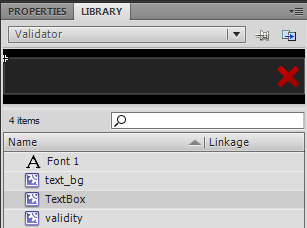Note: If you can't see the library panel you can open it by going to Window > Library or by pressing CTRL+L Using the Text Tool (Hotkey T) create a static text field just above the previously created instance of TextBox and write "Date:" in it.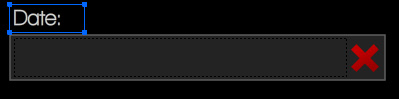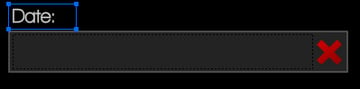Select the ActionScript layer and open the Actions panel by pressing F9 or by going to Window > Actions. Type in the following code in the switch statement from the onFrameUpdate function on line 22:  1 2 case dateField.input_txt:  3  valid = validator.checkDate(input);  4  break;  This will validate the input using the validator instance as a date. Test the movie by pressing CTRL+ENTER on the keyboard or going to Control > Test Movie. Enter a date in the text field in any format(UK or US) using the same separator between the month, day and year. If you entered a correct value the green tick should appear. ## Step 5: E-mail Address Structure An email address represents a reference to the electronic mail box where email messages are sent. The RFC specification states that an email address can be composed from the following: • Uppercase and lowercase English letters (a-z, A-Z) • Digits 0 to 9 • Characters ! #$ % & ' * + - / = ? ^ _  { | } ~ .

Most likely you won't find an email provider which allows all these characters in the email address. To validate such an email address requires a long and very complicated regular expression. As many companies(e.g. Yahoo!, MSN, Gmail) allow only email addresses which contain alphanumeric characters and hyphen "-", dot "." and underscore "_", we will make a checker which we will work with these kind of addresses.

[Ed. Actually, Gmail addresses allow a + sign; we leave it as a challenge for you to implement that ;)]

An email address is usually formed from the "username"(or "address") an "@" sign and the domain(name and extension).

## Step 6: E-mail Address Check

### Code

Paste or type the following code in our Validator class just after the checkDate method:

 1 2 public function checkEmailAddress(emailAddress:String):Boolean  3 {  4  var address:String = "([a-z0-9._-]+)";  5  var domainName:String = "([a-z0-9.-]+)";  6  var domainExt:String = "([a-z]{2,6})";  7   8  var email:RegExp = new RegExp("^" + address + "@" + domainName + "\\." + domainExt + "$", "i");  9   10  return email.test(emailAddress);  11 }  ### Explanation The first line defines the pattern used to check the actual 'address' or username. This checks to see if it contains only the legal characters:  1 var address:String = "([a-z0-9._-]+)";  The second variable defined represents the domain name pattern. A valid domain name and subdomain name contains only alphanumeric characters, the dot '.' and hyphen '-':  1 var domainName:String = "([a-z0-9.-]+)";  Next there is the domain extension pattern used to check if the domain extension is a valid one. A Top Level Domain(TLD) can only contain characters from A to Z, case insensitive with 2 or more characters(in our case 6):  1  var domainExt:String = "([a-z]{2,6})";  To make the check even more strict you can check if the TLD is an existing one:  1  var domainExt:String = "(com|net|org|info|tv|mobi|museum|gov|biz|tel|name|edu|asia|travel|pro)";  These are just a few generic TLDs. You can see how this becomes quite long as you add more and more TLDs. The email variable represents the Regular Expression used to test if the email address is a valid one. This checks if a valid username/address exists at the beginning of the email address, if it has the "a" sign somewhere in the middle after which it checks for a valid domain name and extension both separated by the dot "." Character:  1  var email:RegExp = new RegExp("^" + address + "@" + domainName + "\\." + domainExt + "$", "i"); 

Notice that we haven't used any uppercase letters. This is because we made use of the case-insensitive modifier "i" as generally email addresses are case-insensitive.

## Step 7: E-mail Address Implementation

Note: For more detailed instructions see step 4.

Open Validator.fla, make a new instance of the TextBox movie clip and give it an instance name of emailField.

Create a static Text Field over the TextBox and write in it "E-mail:"

Type the following code on line 25:

 1 2 case emailField.input_txt:  3  valid = validator.checkEmailAddress(input);  4  break; 

This will check the input data in the emailField TextBox as an e-mail address using the validator instance of the Validator class.

Test the movie and enter an e-mail address in the text field labeled with "E-mail".

## Step 8: Web Address Structure

Every time you surf the web you will most likely type a web address (URL) in your web browser to navigate to your desired location. Another place where you might use it is in a comment form where you are asked to type your website (address) or when manually submitting websites to a search engine.

• Protocol or scheme: http, https or ftp + "://"
• Domain name: which can contain any alphanumeric character, dot "." and the hyphen "-"
• Domain extension: made up of alphanumeric characters

## Step 9: Web Address Check

### Code

Paste or type the following code in our Validator class just after the checkEmailAddress method:

 1 2 public function checkWebAddress(address:String):Boolean  3 {  4  var protocol:String = "(https?:\/\/|ftp:\/\/)?";  5  var domainName:String = "([a-z0-9.-]{2,})";  6  var domainExt:String = "([a-z]{2,6})";  7  var web:RegExp = new RegExp('^' + protocol + '?' + domainName + "\." + domainExt + '$', "i");  8   9  return web.test(address);  10 }  ### Explanation The first line defines the pattern used to check the protocol. Usually this can be optional (depending on the case):  1  var protocol:String = "(https?:\/\/|ftp:\/\/)?";  The second variable defined represents the domain name pattern. A valid domain name and subdomain name contains only alphanumeric characters, the dot "." and hyphen "-":  1  var domainName:String = "([a-z0-9.-]{2,})";  Next there is the domain extension pattern used to check if the domain extension is a valid one. A Top Level Domain(TLD) can only contain characters from A to Z, case insensitive with 2 or more characters(in our case 6):  1  var domainExt:String = "([a-z]{2,6})";  To make the check even more strict you can check if the TLD is an existing one:  1  var domainExt:String = "(com|net|org|info|tv|mobi|museum|gov|biz|tel|name|edu|asia|travel|pro)";  These are just a few generic TLDs. You can see how this becomes quite long as you add more and more TLDs. The web variable represents the Regular Expression used to test if the web address is a valid one. This checks if the provided address is made up from the protocol (optional) and domain (the domain must be made up of a domain name and a domain extension separated by the dot "." character:  1  var web:RegExp = new RegExp('^' + protocol + '?' + domainName + "\." + domainExt + '$', "i"); 

As in the case of the e-mail address web addresses are case insensitive so we use the "i"(case insensitive) modifier.

## Step 10: Web Address Implementation

Note: For more detailed instructions see step 4.

Open Validator.fla, make a new instance of the TextBox movie clip and give it an instance name of webAddressField.

Create a static Text Field over the TextBox and write in it "Web address:".

Type the following code on line 28:

 1 2 case webAddressField.input_txt:  3  valid = validator.checkWebAddress(input);  4  break; 

This will check the input data in the webAddressField TextBox as a web address using the validator instance of the Validator class.

Test the movie and enter a web address in the text field labeled with "Web address".

## Step 11: Phone Number Structure

The most common place to use a phone number on the web is a contact form or SMS applications. A phone number is made up of the following two elements:

• Country code: a number between 1 and 999 preceded by a plus "+" sign or double zeros "00" (optional).
• Local phone number: made up of only digits. The number of digits depends on the operator, country and phone number type.

Here you will find a complete list of country calling codes if you would like to restrict your phone number input to only several countries. Also you can check the Numbering Plan to see how telephone numbers are used in different networks and countries.

## Step 12: Phone Number Check

### Code

Paste or type the following code in our Validator class just after the checkWebAddress method:

 1 2 public function checkPhoneNumber(phoneNumber:String):Boolean  3 {  4  var countryCode:String = "((\\+|00)?([1-9]|[1-9][0-9]|[1-9][0-9]{2}))";  5  var num:String = "([0-9]{3,10})";  6  phoneNumber = phoneNumber.match(/[\+\d]/g).join('');  7  var phone:RegExp = new RegExp("^" + countryCode + num +"$");  8   9  return phone.test(phoneNumber);  10 }  ### Explanation The first line defines the pattern used to check the country code. As said this is a number between 1 and 999 preceded by "+" or "00"(optional):  1  var protocol:String = "(https?:\/\/|ftp:\/\/)?";  The second variable represents the actual number. We will make it to check for a number with 3 to 10 digits:  1  var num:String = "([0-9]{3,10})";  On the third line we strip our phone number of any extra spaces or separators keeping only the "+" sign and digits:  1  phoneNumber = phoneNumber.match(/[\+\d]/g).join('');  The phone Regular Expression is the expression used to check if the phone number is made out of a country code and a local number:  1  var phone:RegExp = new RegExp("^" + countryCode + num +"$"); 

The last line returns the result of testing the pattern over the provided phone number. Easy enough, right?

## Step 13: Phone Number Implementation

Note: For more detailed instructions see step 4.

Open Validator.fla, make a new instance of the TextBox movie clip and give it an instance name of phoneNumberField.

Create a static Text Field over the TextBox and write in it "Phone number:".

Type the following code on line 31:

 1 2 case phoneNumberField.input_txt:  3  valid = validator.checkPhoneNumber(input);  4  break; 

This will check the input data in the phoneNumberField TextBox as a phone number using the validator instance of the Validator class.

Test the movie and enter a phone number in the text field labeled with "Phone number".

Well this is what you should have until now:

And this is the code:

 1 2 package  3 {  4  public class Validator  5  {  6   7  public function Validator()  8  {  9  trace('Validator created');  10  }  11   12  public function checkDate(date:String):Boolean  13  {  14  var month:String = "(0?[1-9]|1)";  15  var day:String = "(0?[1-9]|[0-9]|3)";  16  var year:String = "([1-9][0-9]{3})";  17  var separator:String = "([.\/ -]{1})";  18   19  var usDate:RegExp = new RegExp("^" + month + separator + day + "\\2" + year + "$");  20  var ukDate:RegExp = new RegExp("^" + day + separator + month + "\\2" + year + "$");  21   22  return (usDate.test(date) || ukDate.test(date) ? true:false);  23  }  24   25  public function checkEmailAddress(emailAddress:String):Boolean  26  {  27  var address:String = "([a-z0-9._-]+)";  28  var domainName:String = "([a-z0-9.-]+)";  29  var domainExt:String = "(com|net|org|info|tv|mobi|museum|gov|biz|tel|name|edu|asia|travel|pro)";  30   31  var email:RegExp = new RegExp("^" + address + "@" + domainName + "\\." + domainExt + "$", "i");  32   33  return email.test(emailAddress);  34  }  35   36  public function checkWebAddress(address:String):Boolean  37  {  38  var protocol:String = "(https?:\/\/|ftp:\/\/)?";  39  var domainName:String = "([a-z0-9.-]{2,})";  40  var domainExt:String = "(com|net|org|info|tv|mobi|museum|gov|biz|tel|name|edu|asia|travel|pro)";  41  var web:RegExp = new RegExp('^' + protocol + '?' + domainName + "\\." + domainExt + '$', "i");  42   43  return web.test(address);  44  }  45   46  public function checkPhoneNumber(phoneNumber:String):Boolean  47  {  48  var countryCode:String = "((\\+|00)?([1-9]|[1-9][0-9]|[1-9][0-9]{2}))";  49  var num:String = "([0-9]{3,10})";  50  phoneNumber = phoneNumber.match(/[\+\d]/g).join('');  51  var phone:RegExp = new RegExp("^" + countryCode + num +"$");  52   53  return phone.test(phoneNumber);  54  }  55   56  }  57 58 }  Compare your results with mine and make sure everything is where is supposed to be. Now... let's move on! ## Step 14: ISBN-10 Structure International Serial Book Number, or for short ISBN, is an international identification number given to a book. ISBN numbers are unique and are only give once to a book. Until now we couldn't check if the data was actually valid but ISBN numbers have a control digit which needs to be verified with a checksum algorithm. ### The Checksum Algorithm: • We strip the number of slashes or spaces and store the last digit separately (this is the control digit). • We multiply every digit in the 9 digits number with his weight. The weights range from 10 to 2 starting from the first digit of the number.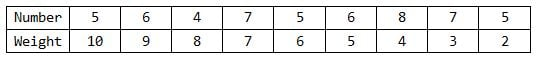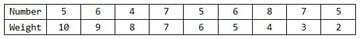• We sum the resulted numbers and we extract the remainder for the division of the number with 11. • If the remainder is 0 the control character must be 0. If the remainder is not 0 we substract it from 11 and if the result is 10 the control character must be "x" else the control character must match the result. If you need more detailed specifications about ISBN numbers you can find all about them Here. The wiki page contains details about both ISBN-10 and ISBN-13 numbers. ## Step 15: ISBN-10 Check ### Code Paste or type the following code in our Validator class just after the checkPhoneNumber method:  1 2 public function validateISBN10(isbn10:String):Boolean  3 {  4  isbn10 = isbn10.replace(/[ -]/g, '');  5   6  if (isbn10.length != 10)  7  {  8  return false;  9  }else  10  {  11  var valid:Boolean;  12  var weights:Array = [10, 9, 8, 7, 6, 5, 4, 3, 2];  13  var digits:Array = isbn10.split('');  14  var control:String = digits.pop();  15  var result:uint = 0;  16   17  for (var i:uint = 0; i < 9; i++)  18  {  19  digits[i] = digits[i] * weights[i];  20  result += digits[i];  21  }  22  result = (result%11==0)?0:(11 - result % 11);  23  switch(result)  24  {  25  case 10:  26  valid = (control.toLowerCase() == 'x');  27  break;  28  default:  29  valid = control == String(result);  30  break;  31  }  32  return valid;  33  }  34 }  ### Explanation The first lines in our method strip the number of any spaces and hyphens. Then it checks if the length is correct. An ISBN-10 number must have 9 digits + a control character:  1 2 isbn10 = isbn10.replace(/[ -]/g, '');  3   4 if (isbn10.length != 10)  5 {  6  return false;  7 }  In the else the variable valid stores the validity of the number and weights is an array with the weights of the digits:  1 2 var valid:Boolean;  3 var weights:Array = [10, 9, 8, 7, 6, 5, 4, 3, 2];  In the digits array we store the digits of the number and extract the last character which is the control character and store it in the control variable. The result variable will store the result of the check sum:  1 2 var digits:Array = isbn10.split('');  3 var control:String = digits.pop();  4 var result:uint = 0;  Now that we have every digit in the number we must multiply it by its weight. We can do this with a for-loop in which we multiply every element (digit) in the digits array with its corresponding weight and store the sum of these multiplications:  1 2 for (var i:uint = 0; i < 9; i++)  3 {  4  digits[i] = digits[i] * weights[i];  5  result += digits[i];  6 }  In the next line of code we check the remainder of the sum with respect to 11:  1 result = (result%11==0)?0:(11 - result % 11);  This could have been done with an if statement but I've used the ternary operator which is a shorthand method. The ternary operator works like so variable = condition ? value1 : value2;. If the condition is true value1 will be given to the variable else it will receive value2. Next we check the result with a switch statement and give the value to the valid variable. If it's 10 then the the control character must be "x" else the result must match the corresponding digit:  1 2 switch(result)  3 {  4  case 10:  5  valid = (control.toLowerCase() == 'x');  6  break;  7  default:  8  valid = control == String(result);  9  break;  10 }  And lastly we return the result of the test. ## Step 16: ISBN-10 Implementation Note: For more detailed instructions see step 4. Open Validator.fla, make a new instance of the TextBox movie clip and give it an instance name of ISBNField. Create a static Text Field over the TextBox and write in it "ISBN number:". Type the following code on line 34:  1 2 case ISBNField.input_txt:  3 valid = validator.validateISBN10(input);  4  break;  This will check the input data in the ISBNField TextBox as a ISBN-10 using the validator instance of the Validator class. Test the movie and enter a ISBN-10 number in the text field labeled with "ISBN number". Be aware that validating an ISBN-10 number doesn't necessarily mean that this exists or belongs to any book. ## Step 17: ISBN-13 Structure "What? Another ISBN number?" you might say. Well, actually the previous number format is an old standard. This new ISBN-13 standard was introduced in 2005 and until 01.01.2007 the both formats were used for books. The ISBN-13 format has 12 digits and a thirteenth digit being the control digit. ### The Checksum Algorithm: • We strip the number of spaces and hyphens. • We multiply every digit with its corresponding weight. The weights are 1 and 3 and are distributed like so: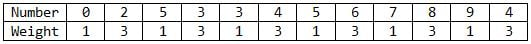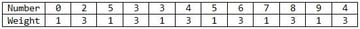• We sum the resulted numbers and we extract the remainder for the division of the number with 10. • If the remainder is 0 the control character must be 0. If the remainder is not 0 we substract it from 10 and the result must match the control character. ## Step 18: ISBN-13 Check ### Code Paste or type the following code in our Validator class just after the validateISBN10 method:  1 2 public function validateISBN13(isbn13:String):Boolean  3 {  4  var digits:Array = isbn13.match(/\d/g);  5  var control:uint = digits.pop();  6  var result:uint;  7  var weight:uint;  8  if (digits.length != 12)  9  {  10  return false;  11  }else {  12  for (var i:uint = 0; i < 12; i++)  13  {  14  weight = (i % 2 == 0)?1:3;  15  digits[i] = digits[i] * weight;  16  result += digits[i];  17  }  18  result = (result % 10 == 0)?0:(10 - result % 10);  19  return (result == control);  20  }  21 }  ### Explanation The digits array contains the digits of the number. We do this by extracting the digits from our number using the match method of the String class. After that we store the control digit in the control variable by extracting the last element in the digits array:  1 2 var digits:Array = isbn13.match(/\d/g);  3 var control:uint = digits.pop();  The weight variable will hold the current weight of a digit and the result variable will hold the result of the checksum:  1 2 var weight:uint;  3 var result:uint;  We use an in-else statement to see if there is the correct number of digits in the digits array, excluding the control digit. In the for-loop we iterate through the digits array and multiply every digit with its weight (1 and 3 alternating them every digit) and store the sum in the result variable. If the position of the digit in the array is an even number the weight is 1 else the weight is 3. We do this by checking the remainder for the division of i by 2:  1 2 weight = (i % 2 == 0)?1:3;  3 digits[i] = digits[i] * weight;  4 result += digits[i];  Lastly we make division by 10 of the sum. If it's 0 the result is 0 else we substract the remainder from 10:  1 2 result = (result % 10 == 0)?0:(10 - result % 10);  3 return (result == control);  If the result digit is the same as the control digit the number is valid. ## Step 19: ISBN-13 Implementation Open Validator.fla and modify the code on lines 34 to 36 like so:  1 2 case ISBNField.input_txt:  3 valid = validator.validateISBN10(input)||validator.validateISBN13(input);  4  break;  This will check the number both as ISBN-10 and ISBN-13. Test the movie and enter an ISBN number in the text field labeled with "ISBN number". The same goes for this type of number as in the case of ISBN-10 ones. If a number is valid it doesn't necessarily mean that it also exists. ## Step 20: IBAN Structure International Bank Account Number or IBAN for short is an international standard for numbering bank accounts. This is made up of the following: • Two alphabetic characters (uppercase) representing the country • A check number made up of two digits ranging from 00 to 99 • Four alphabetic characters (uppercase) representing the bank who issued the number • An account number of various lengths depending on the country and bank ### The Validation Algorithm: • We strip the number of spaces. • The first four characters in the number (country characters and control digits) are moved to the back of the number. • We convert all alphabetic characters in numbers like so: A = 10, B = 11, C = 12, ..., Z = 35. • We compute the remainder of the division by 97. The number is valid only if the remainder is 1. Check this page for a complete documentation on International Bank Account Numbers. ## Step 21: IBAN Check ### Code Paste or type the following code in our Validator class just after the validateISBN13 method:  1 2 public function validateIBAN(iban:String):Boolean  3 {  4  var nums:Object = { A:10, B:11, C:12, D:13, E:14,  5  F:15, G:16, H:17, I:18, J:19,  6  K:20, L:21, M:22, N:23, O:24,  7  P:25, Q:26, R:27, S:28, T:29,  8  U:30, V:31, W:32, X:33, Y:34, Z:35 };  9  var chars:Array = iban.split('');  10   11  for (var i:int = 0; i < 4; i++)  12  {  13  chars.push(chars.shift());  14  }  15   16  var exp:RegExp = /[a-z]/i;  17  for (var j:int = 0; j < chars.length; j++)  18  {  19  chars[j] = exp.test(chars[j]) ? nums[chars[j].toUpperCase()] : chars[j];  20  }  21  iban = chars.join('');  22  return modulus(iban, 97) == 1;  23 }  ### Explanation The nums object contains the corresponding number for each character:  1 2 var nums:Object = { A:10, B:11, C:12, D:13, E:14,  3  F:15, G:16, H:17, I:18, J:19,  4  K:20, L:21, M:22, N:23, O:24,  5  P:25, Q:26, R:27, S:28, T:29,  6  U:30, V:31, W:32, X:33, Y:34, Z:35 };  The chars array contains the characters in the number:  1  var chars:Array = iban.split('');  With the first for-loop we move the first four characters to the back by removing them from the beginning of the array and placing them to the back:  1 2 for (var i:int = 0; i < 4; i++)  3 {  4  chars.push(chars.shift());  5 }  The exp regular expression is a simple pattern for alphabetic characters used to test every element in the chars array:  1  var exp:RegExp = /[a-z]/i;  In the second for-loop we check every character in the chars array to see if it's a letter and change it to its corresponding number using the nums object:  1 2 for (var j:int = 0; j < chars.length; j++)  3 {  4  chars[j] = exp.test(chars[j]) ? nums[chars[j].toUpperCase()] : chars[j];  5 }  After converting every letter in a number we transform the array into a string and assign it to the iban variable:  1  iban = chars.join('');  Lastly we return if the remainder of the division by 97 of the number is equal to 1 or not.  1  return modulus(iban, 97) == 1;  You might wonder why I've used a custom function instead of the "%" operator. Well… if you think about it, the number has 20+ digits (very large) and the normal modulus operator will not work as expected. So we need to define a way to calculate the modulus for large numbers. ### The Modulus Function To calculate the modulus of large numbers I've used an implementation of the "divide and conquer" method. To keep it short this method substracts a part of the large number and makes the modulus of that part after which it appends the result to the remaining portion of the large number. For a more detailed presentation of this method see This link. Paste or type the following code in our Validator class just after the validateISBN method:  1 2 public function modulus(largeNumber:String, mod:uint):Number  3 {  4  var tmp:String = largeNumber.substr(0, 10);  5  var number:String = largeNumber.substr(tmp.length);  6  var result:String;  7   8  do {  9  result = String(Number(tmp) % mod);  10  number = result + number;  11  tmp = number.substr(0, 10);  12  number = number.substr(tmp.length);  13   14  } while (number.length > 0);  15   16  return Number(tmp) % mod;  17 }  The tmp variable stores a part of the large number by substracting 10 characters from it:  1  var tmp:String = largeNumber.substr(0, 10);  The number variable represents the large number which gets trimmed by the length of the tmp string:  1  var number:String = largeNumber.substr(tmp.length);  The result variable will obviously hold the result of the division. In the do-while loop we compute the remainder for the division of the tmp number by the mod integer (the divider) and assign the value to the result:  1 result = String(Number(tmp) % mod);  We add the result to the beginning of the large number and repeat the previously three steps while the number length is larger than 0:  1 2 number = result + number;  3 tmp = number.substr(0, 10);  4 number = number.substr(tmp.length);  And lastly we return the result of the modulus. ## Step 22: IBAN Implementation Note: For more detailed instructions see step 4. Open Validator.fla, make a new instance of the TextBox movie clip and give it an instance name of IBANField. Create a static Text Field over the TextBox and write in it "IBAN number:". Type the following code on line 37:  1 2 case IBANField.input_txt:  3 valid = validator.validateIBAN(input);  4  break;  This will check the input data in the IBANField TextBox as an IBAN using the validator instance of the Validator class. Test the movie and enter an IBAN number in the text field labeled with "IBAN number". ## Step 23: Card Number Structure On the front of every credit or debit card you will find a number which varies in length. You use this number usually to make online payments using your credit card. As I said, the number varies in length and format depending on the company which it belongs to. But they all have one thing in common: they must pass a checksum algorithm. Credit cards numbers are validated using the Luhn (mod 10) algorithm. Before we dive into the actual validation we need to check if the number belongs to any valid company. We will check for these three main card issuers: American Express, Dinners Club, MasterCard and Visa. The following table contains the number details for each company: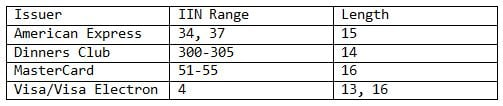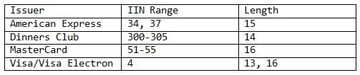The IIN Range is the number which the card number begins with. ### The Luhn Algorithm • We double every second digit in the number starting from the first digit if the number has an even number of digits or starting from the second digit otherwise. • We sum the digits of the product together with the undoubled digits. • We check if the total sum modulo 10 is equal to 0 (or the number ends in 0) the number is valid. For further reading see the Bank Card Number and the Luhn Algorithm wiki pages ## Step 24: Card Number Check ### Code Paste or type the following code in our Validator class just after the validateIBAN method:  1 2 public function validateCardNumber(ccNumber:String):Boolean  3 {  4  var americanExpress:RegExp = /^(34|37) ([0-9]{13})$/x;  5  var dinnersClub:RegExp = /^(30[0-5]) ([0-9]{13})$/x;  6  var masterCard:RegExp = /^(5[1-5]) ([0-9]{14})$/x;  7  var visa:RegExp = /^4 ([0-9]{12} | [0-9]{15})$/x;  8  var valid:Boolean;  9  ccNumber = ccNumber.match(/\d/g).join('');  10   11  if (americanExpress.test(ccNumber) || dinnersClub.test(ccNumber) ||  12  masterCard.test(ccNumber) || visa.test(ccNumber))  13  valid = true;  14   15   16  return valid && luhnChecksum(ccNumber);  17 }  ### Explanation The americanExpress regular expression defines the pattern used to check American Express card numbers. This will check for numbers beginning with 34 or 37 and has 13 more digits:  1  var americanExpress:RegExp = /^(34|37) ([0-9]{13})$/x; 

The dinnersClub regular expression defines the pattern used to check Dinners Club card numbers. This pattern checks if a credit card number begins with a number ranging from 300 to 305 and has 13 more digits:

 1  var dinnersClub:RegExp = /^(30[0-5]) ([0-9]{13})$/x;  The masterCard regular expression defines the patter for MasterCard card numbers. This pattern will check if the credit card number begins with a number between 51 and 55 and ends with 14 more digits:  1  var masterCard:RegExp = /^(5[1-5]) ([0-9]{14})$/x; 

The visa regular expression defines the pattern for Visa/Visa Electron card numbers. This will check if the provided card number begins with 4 and ends with 12 or 15 mode digits:

 1  var visa:RegExp = /^4 ([0-9]{12} | [0-9]{15})$/x;  We use the extended modifier ("x") in every expression so that whitespaces will be ignored from. This is used just to make the expression easier to read. The Boolean variable valid will hold the validity status of the card number. On the sixth line we extract the digits from our number (the number is usually written with hyphens or spaces for readability purposes):  1  ccNumber = ccNumber.match(/\d/g).join('');  In the if statement we check if the number matches any of the four patterns defined earlier:  1   2 if (americanExpress.test(ccNumber) || dinnersClub.test(ccNumber) ||  3  masterCard.test(ccNumber) || visa.test(ccNumber))  4  valid = true;  On the last line we return true if the number is valid and passes the Luhn checksum. ### The Luhn Algorithm Type in or paste the following code after the validateCardNumber method in our Validator class:  1 2 public function luhnChecksum(number:String):Boolean  3 {  4  var digits:Array = number.split('');  5  var start:uint = (number.length % 2 == 0) ? 0:1;  6  var sum:int;  7   8  while (start < digits.length)  9  {  10  digits[start] = uint(digits[start]) * 2;  11  start += 2;  12  }  13   14  digits = digits.join('').split('');  15   16  for (var i:uint = 0; i < digits.length; i++)  17  {  18  sum += uint(digits[i]);  19  }  20  return (sum % 10 == 0);  21 }  The digits variable contains the digits of the number. The start variable is used to define from which digit to start doubling (this is represented by the index in the digits array). The sum variable holds the total sum. We use the while loop to double every second digit in the array. We also add 2 to the start variable to skip at every second digit in the array:  1 2 while (start < digits.length)  3 {  4  digits[start] = uint(digits[start]) * 2;  5  start += 2;  6 }  On the next line we transform the digits array into a string and split it again into an array. We do this because if the doubled numbers are bigger than 9 we must sum the digits (e.g. for 12 we do 1+2):  1  digits = digits.join('').split('');  In the for-loop we simply sum all the digits:  1 2 for (var i:uint = 0; i < digits.length; i++)  3 {  4  sum += uint(digits[i]);  5 }  And lastly we check if the remainder is 0 and return true or false otherwise:  1  return (sum % 10 == 0);  ## Step 25: Card Number Implementation Note: For more detailed instructions see step 4. Open Validator.fla, make a new instance of the TextBox movie clip and give it an instance name of cardNumberField. Create a static Text Field over the TextBox and write in it "Card number:". Type the following code on line 40:  1 2 case cardNumberField.input_txt:  3  valid = validator.validateCreditCardNumber(input);  4  break;  This will check the input data in the cardNumberField TextBox as a Card Number using the validator instance of the Validator class. Test the movie and enter a Card Number number in the text field labeled with "Card number". ## Final code Now this is how our final class looks:  1 2 package  3 {  4  public class Validator  5  {  6   7  public function Validator()  8  {  9  trace('Validator created');  10  }  11  /**  12  * Validates a date in these two formats:  13  *  14  * DD MM YYYY  15  * MM DD YYYY  16  *  17  * The valid separators are dash "-", dot ".", front slash "/" and space " ".  18  *  19  * @param date The date to be validated.  20  * @return Returns true if the date is valid or false otherwise.  21  */  22  public function checkDate(date:String):Boolean  23  {  24  var month:String = "(0?[1-9]|1)";  25  var day:String = "(0?[1-9]|[0-9]|3)";  26  var year:String = "([1-9][0-9]{3})";  27  var separator:String = "([.\/ -]{1})";  28   29  var usDate:RegExp = new RegExp("^" + month + separator + day + "\\2" + year + "$");  30  var ukDate:RegExp = new RegExp("^" + day + separator + month + "\\2" + year + "$");  31   32  return (usDate.test(date) || ukDate.test(date) ? true:false);  33  }  34  /**  35  * Validates an email address. The address should have the following  36  * format:  37  *  38  * [user]@[domain].[domain_extension]  39  *  40  * @param emailAddress  41  * @return Returns true if the address is valid or false otherwise.  42  */  43  public function checkEmailAddress(emailAddress:String):Boolean  44  {  45  var address:String = "([a-z0-9._-]+)";  46  var domainName:String = "([a-z0-9.-]+)";  47  var domainExt:String = "(com|net|org|info|tv|mobi|museum|gov|biz|tel|name|edu|asia|travel|pro)";  48   49  var email:RegExp = new RegExp("^" + address + "@" + domainName + "\\." + domainExt + "$", "i");  50   51  return email.test(emailAddress);  52  }  53  /**  54  * Validates a web address. The address should have the following  55  * format:  56  *  57  * [protocol://(optional)][domain].[domain_extension]  58  *  59  * @param address The web address to be checked.  60  * @return Returns true if the address is valid or false otherwise.  61  */  62  public function checkWebAddress(address:String):Boolean  63  {  64  var protocol:String = "(https?:\/\/|ftp:\/\/)?";  65  var domainName:String = "([a-z0-9.-]{2,})";  66  var domainExt:String = "(com|net|org|info|tv|mobi|museum|gov|biz|tel|name|edu|asia|travel|pro)";  67  var web:RegExp = new RegExp('^' + protocol + '?' + domainName + "\." + domainExt + '$', "i");  68   69  return web.test(address);  70  }  71  /**  72  * Validates a phone number. The phone number should have the following  73  * format:  74  *  75  * [countryCode(optional)][XXX][YYY][ZZZZ]  76  *  77  * Separators between X's, Y's and Z's are optional.  78  * Valid separators are dash "-", dot "." and space " ".  79  *  80  * @param phoneNumber The phone number to be checked.  81  * @return Returns true if the number is valid or false otherwise.  82  */  83  public function checkPhoneNumber(phoneNumber:String):Boolean  84  {  85  var countryCode:String = "((\\+|00)?([1-9]|[1-9][0-9]|[1-9][0-9]{2}))";  86  var num:String = "([0-9]{3,10})";  87  phoneNumber = phoneNumber.match(/[\+\d]/g).join('');  88   89  var phone:RegExp = new RegExp("^" + countryCode + num +"$");  90   91  return phone.test(phoneNumber);  92  }  93  /**  94  * Checks if an ISBN-10 number passes the checksum.  95  *  96  * @param isbn10 The ISBN-10 number to be validated.  97  * @return Returns true if the number is valid or false otherwise.  98  */  99  public function validateISBN10(isbn10:String):Boolean  100  {  101  isbn10 = isbn10.replace(/[ -]/g, '');  102   103  if (isbn10.length != 10)  104  {  105  return false;  106  }else  107  {  108  var valid:Boolean;  109  var weights:Array = [10, 9, 8, 7, 6, 5, 4, 3, 2];  110  var digits:Array = isbn10.split('');  111  var control:String = digits.pop();  112  var result:uint = 0;  113   114  for (var i:uint = 0; i < 9; i++)  115  {  116  digits[i] = digits[i] * weights[i];  117  result += digits[i];  118  }  119  result = (result%11==0)?0:(11 - result % 11);  120  switch(result)  121  {  122  case 10:  123  valid = (control.toLowerCase() == 'x');  124  break;  125  default:  126  valid = control == String(result);  127  break;  128  }  129  return valid;  130  }  131  }  132  /**  133  * Checks the format of an ISBN-13 number and validates it.  134  *  135  * @param isbn13 The ISBN-13 number to be validated.  136  * @return Returns true if the number is valid or false otherwise.  137  */  138  public function validateISBN13(isbn13:String):Boolean  139  {  140  var digits:Array = isbn13.match(/\d/g);  141  var control:uint = digits.pop();  142  var result:uint;  143  var weight:uint;  144  if (digits.length != 12)  145  {  146  return false;  147  }else {  148  for (var i:uint = 0; i < 12; i++)  149  {  150  weight = (i % 2 == 0)?1:3;  151  digits[i] = digits[i] * weight;  152  result += digits[i];  153  }  154  result = (result % 10 == 0)?0:(10 - result % 10);  155  return (result == control);  156  }  157  }  158  /**  159  * Validates an IBAN number.  160  *  161  * @param iban The IBAN number to be validated.  162  * @return Returns true if the number is valid or false otherwise.  163  */  164  public function validateIBAN(iban:String):Boolean  165  {  166  var nums:Object = { A:10, B:11, C:12, D:13, E:14,  167  F:15, G:16, H:17, I:18, J:19,  168  K:20, L:21, M:22, N:23, O:24,  169  P:25, Q:26, R:27, S:28, T:29,  170  U:30, V:31, W:32, X:33, Y:34, Z:35 };  171  var chars:Array = iban.split('');  172   173  for (var i:int = 0; i < 4; i++)  174  {  175  chars.push(chars.shift());  176  }  177   178  var exp:RegExp = /[a-z]/i;  179  for (var j:int = 0; j < chars.length; j++)  180  {  181  chars[j] = exp.test(chars[j]) ? nums[chars[j].toUpperCase()] : chars[j];  182  }  183  iban = chars.join('');  184  return modulus(iban, 97) == 1;  185  }  186  /**  187  * Checks if the provided Credit Card number is a correct one for each  188  * of these providers: American Express, Dinners Club, MasterCard and Visa.  189  *  190  * @param ccNumber The credit number to be validated.  191  * @return Returns true if the number is valid or false otherwise  192  */  193  public function validateCardNumber(ccNumber:String):Boolean  194  {  195  var americanExpress:RegExp = /^(34|37) ([0-9]{13})$/x;  196  var dinnersClub:RegExp = /^(30[0-5]) ([0-9]{13})$/x;  197  var masterCard:RegExp = /^(5[1-5]) ([0-9]{14})$/x;  198  var visa:RegExp = /^4 ([0-9]{12} | [0-9]{15})$/x;  199  var valid:Boolean;  200  ccNumber = ccNumber.match(/\d/g).join('');  201   202  if (americanExpress.test(ccNumber) || dinnersClub.test(ccNumber) ||  203  masterCard.test(ccNumber) || visa.test(ccNumber))  204  valid = true;  205   206   207  return valid && luhnChecksum(ccNumber);  208  }  209  /**  210  * Returns the modulus of a very large number.  211  *  212  * @param largeNumber The divided number.  213  * @param mod The dividing number.  214  *  215  * @return Returns the remainder.  216  */  217  public function modulus(largeNumber:String, mod:uint):Number  218  {  219  var tmp:String = largeNumber.substr(0, 10);  220  var number:String = largeNumber.substr(tmp.length);  221  var result:String;  222   223  do {  224  result = String(Number(tmp) % mod);  225  number = result + number;  226  tmp = number.substr(0, 10);  227  number = number.substr(tmp.length);  228   229  } while (number.length > 0);  230   231  return Number(tmp) % mod;  232  }  233  /**  234  * Makes a Luhn mod 10 checksum for a specified number.  235  *  236  * @param number The number to be checked.  237  * @return Returns true if the number passes the checksum or false otherwise.  238  */  239  public function luhnChecksum(number:String):Boolean  240  {  241  var digits:Array = number.split('');  242  var start:uint = (number.length % 2 == 0) ? 0:1;  243  var sum:int;  244   245  while (start < digits.length)  246  {  247  digits[start] = uint(digits[start]) * 2;  248  start += 2;  249  }  250   251  digits = digits.join('').split('');  252   253  for (var i:uint = 0; i < digits.length; i++)  254  {  255  sum += uint(digits[i]);  256  }  257  return (sum % 10 == 0);  258  }  259  }  260 261 } `

I've added comments to the final result so that you remember how each method works and how the tested value should look like.

You can use this class in any of your AS3 projects.

## Conclusion

Remember that even if you've validated your data this might not be real (as in the case of ISBNs, email addresses, IBANs etc) as many of these can be generated or random. But checking the data before registering it to your database, sending emails or submiting it to a payment server might catch some typos and get the user on the right track.

I hope this tutorial about various validations in ActionScript has helped you to understand the basics of input data validation. Next you can try to modify these methods and make them more restrictive, or more specific to your needs. Also you can try to make your own validation methods for any other data.